Ex 4.2

Chapter 4 Class 7 Simple Equations
Serial order wise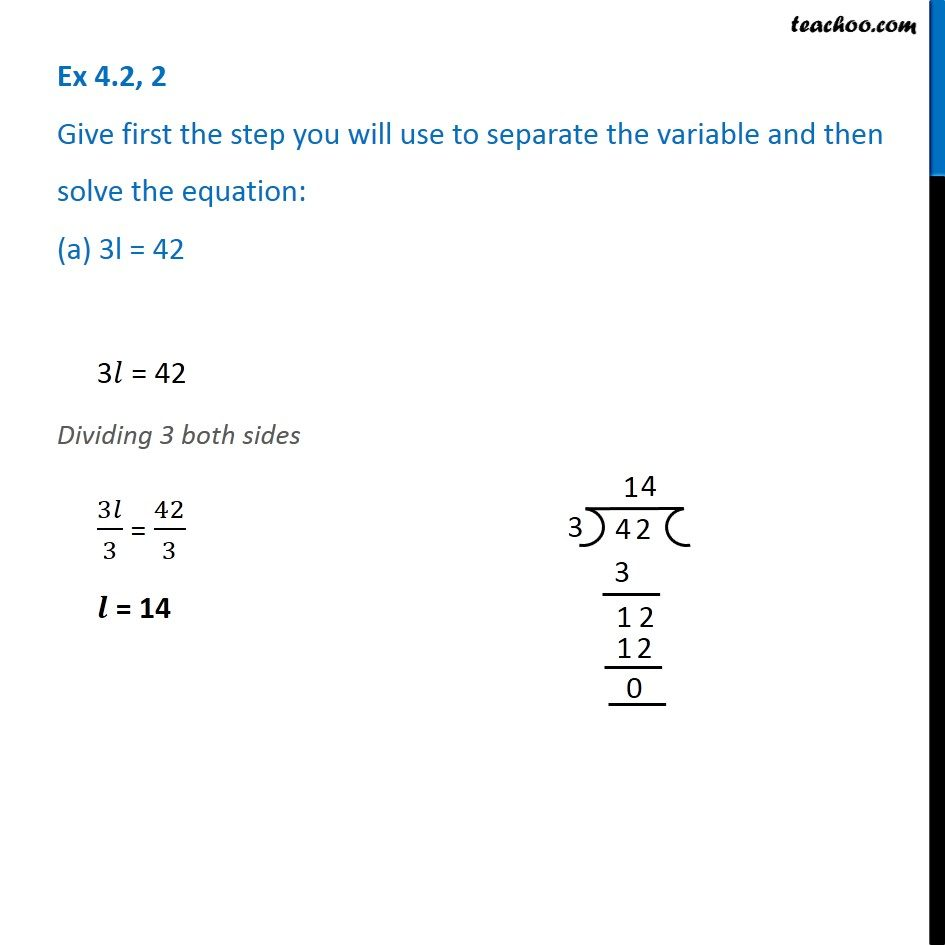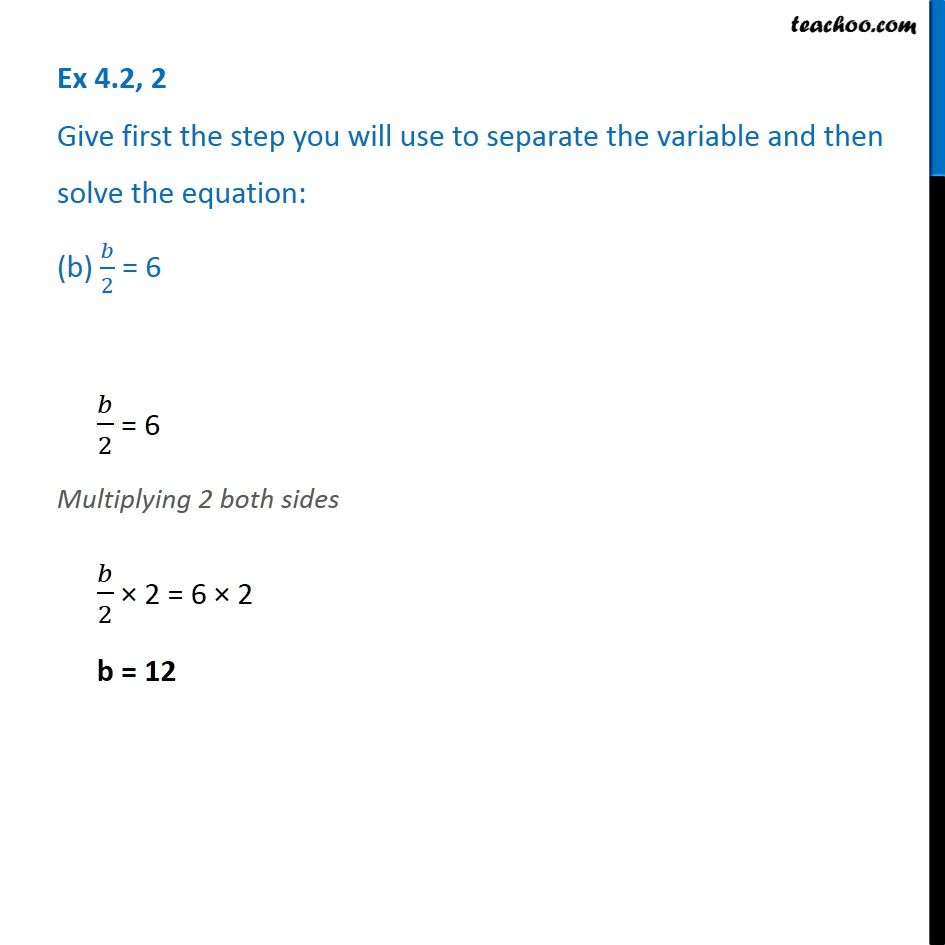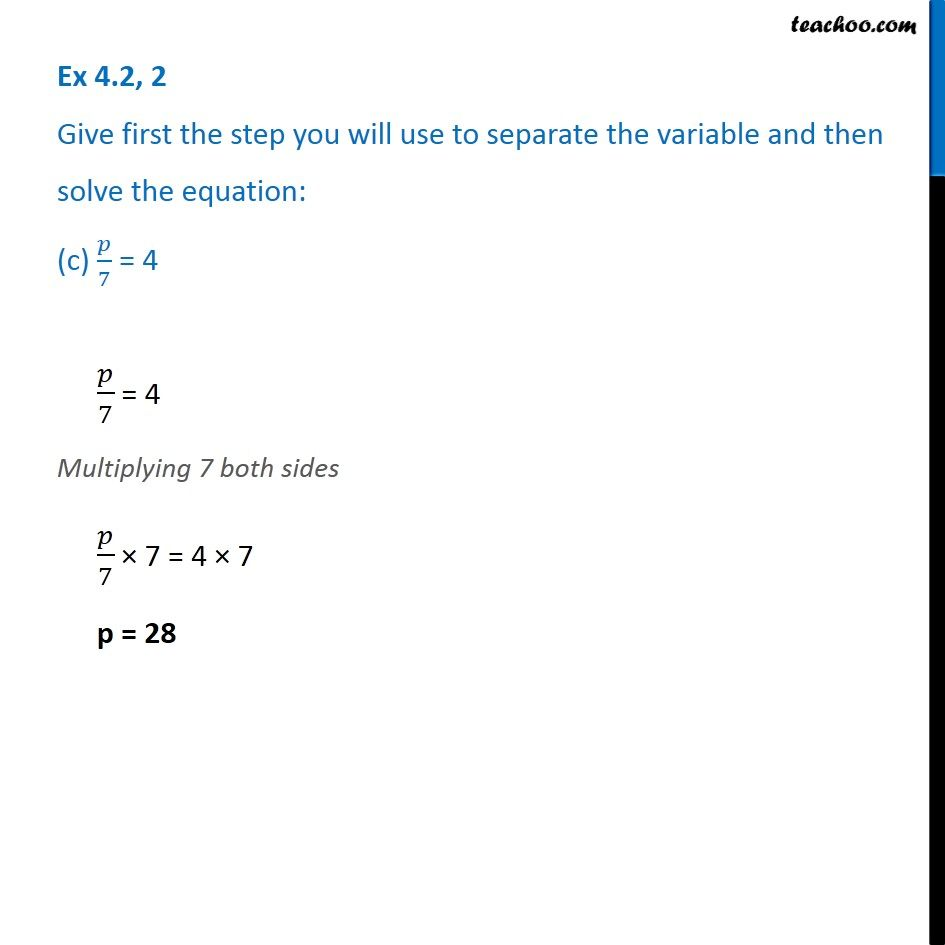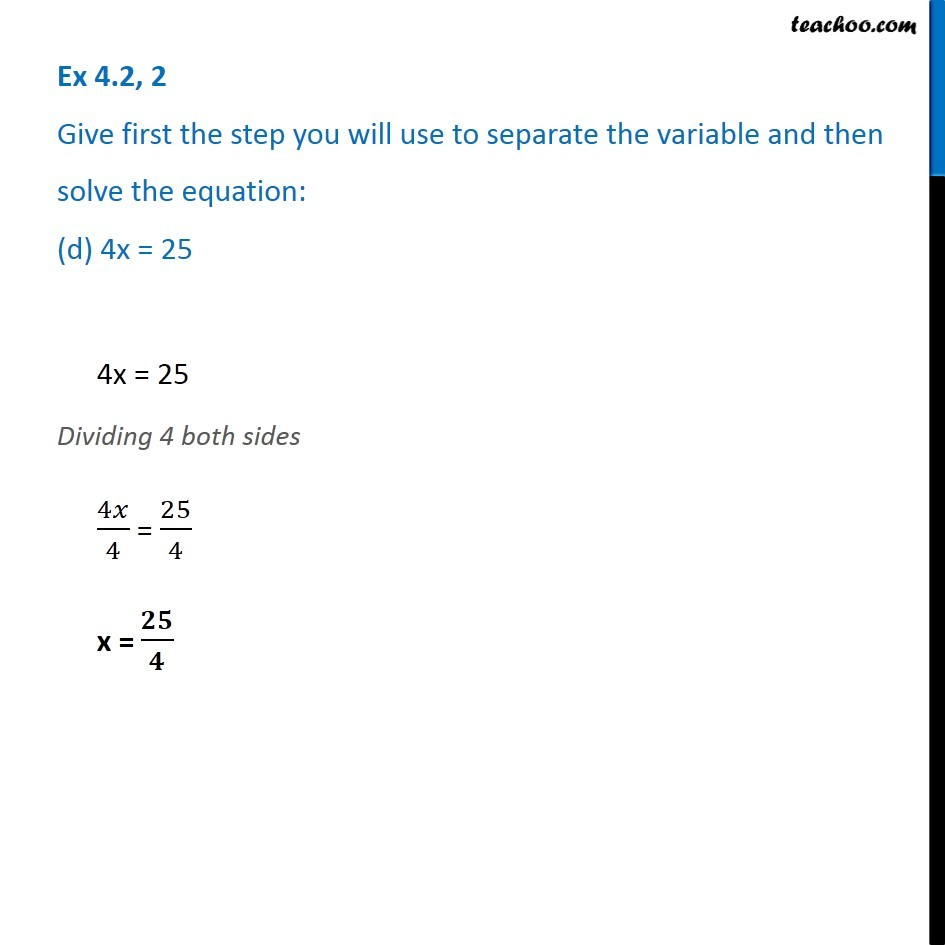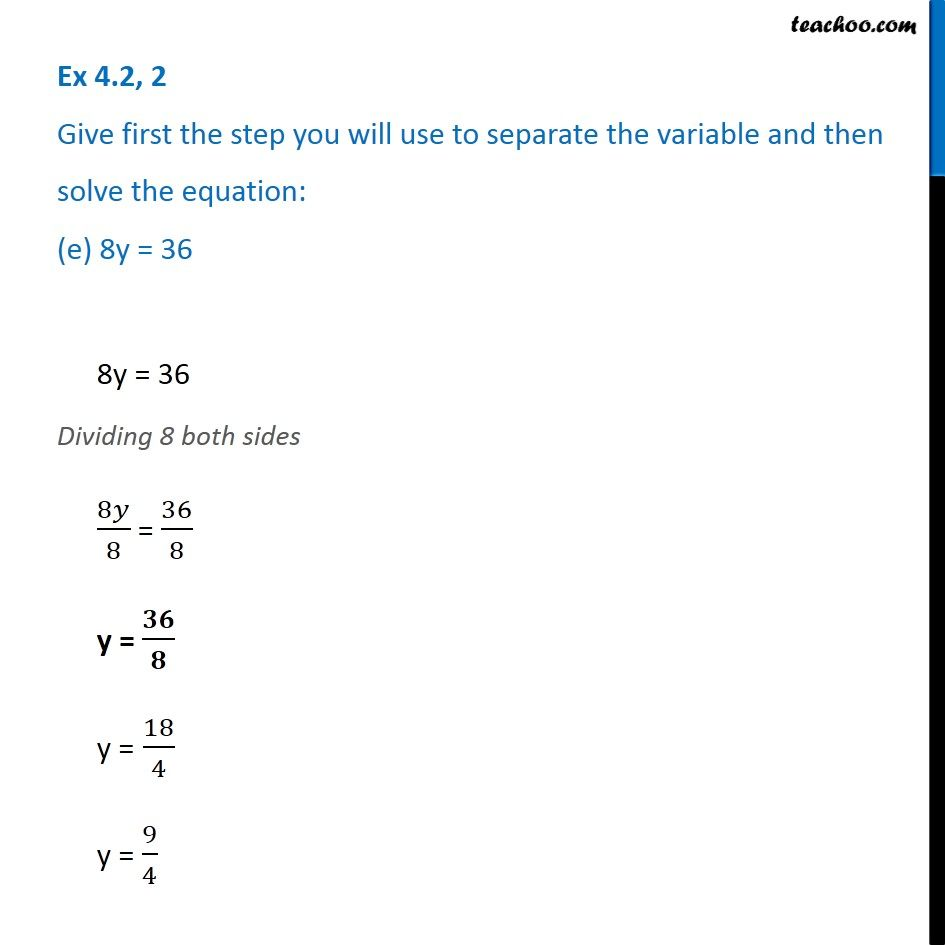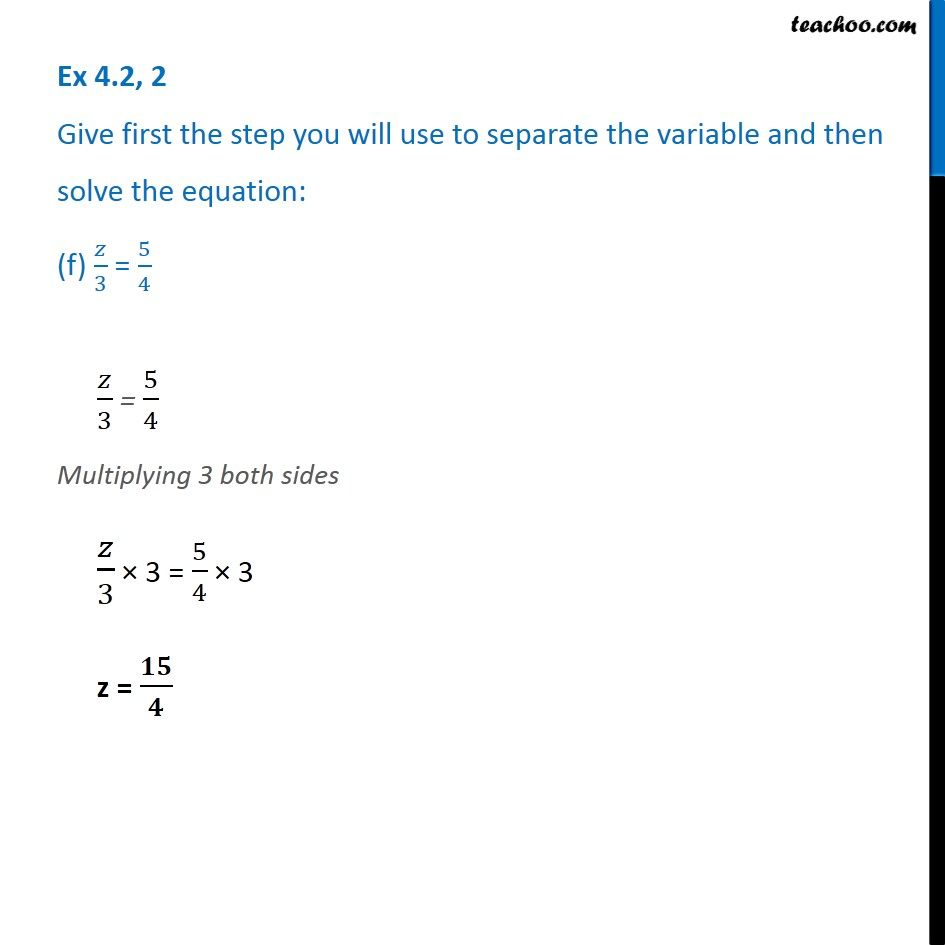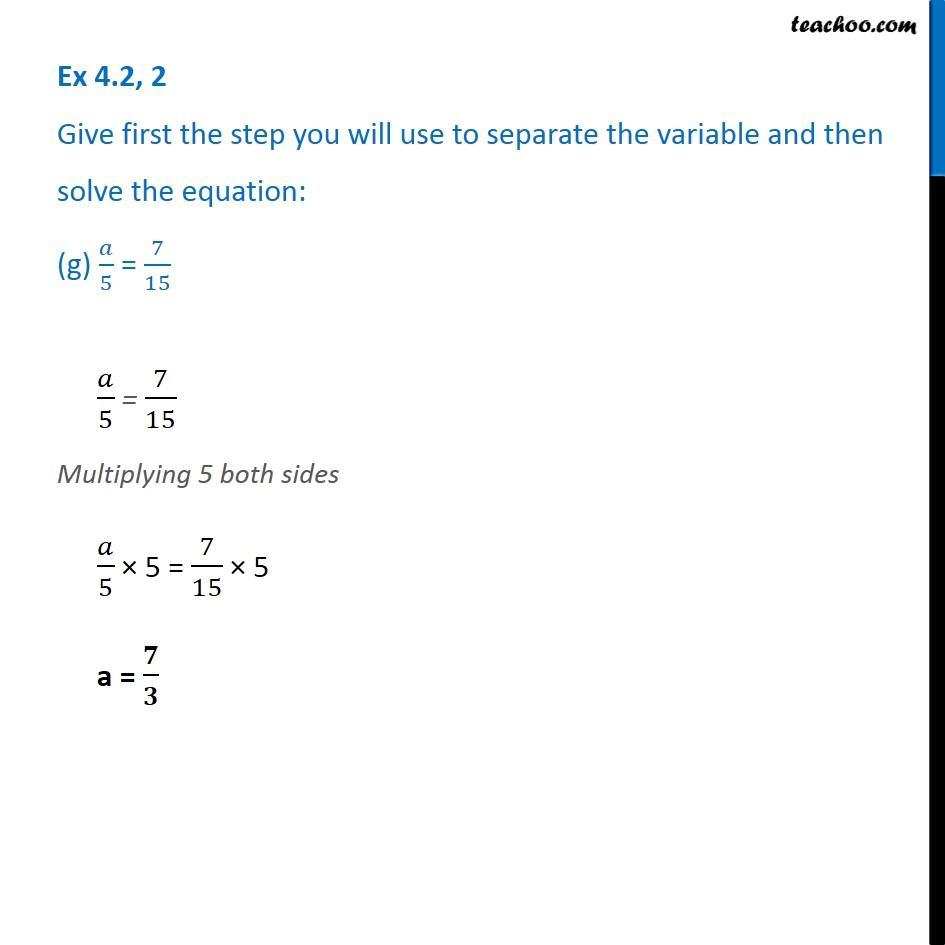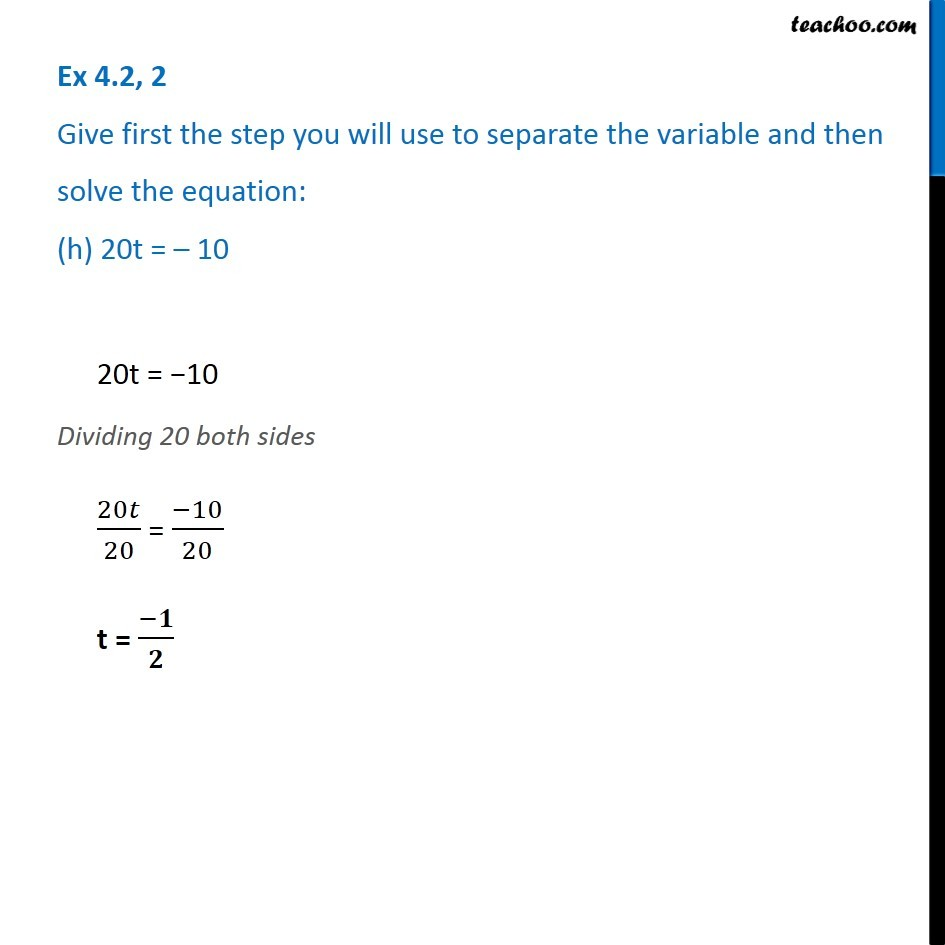Learn in your speed, with individual attention - Teachoo Maths 1-on-1 Class

### Transcript

Ex 4.2, 2 Give first the step you will use to separate the variable and then solve the equation: (a) 3l = 423𝑙 = 42 Dividing 3 both sides 3𝑙/3 = 42/3 𝒍 = 14 Ex 4.2, 2 Give first the step you will use to separate the variable and then solve the equation: (b) 𝑏/2 = 6𝑏/2 = 6 Multiplying 2 both sides 𝑏/2 × 2 = 6 × 2 b = 12 Ex 4.2, 2 Give first the step you will use to separate the variable and then solve the equation: (d) 4x = 25 Ex 4.2, 2 Give first the step you will use to separate the variable and then solve the equation: (e) 8y = 36 Dividing 8 both sides 8𝑦/8 = 36/8 y = 𝟑𝟔/𝟖 y = 18/4 y = 9/4 Ex 4.2, 2 Give first the step you will use to separate the variable and then solve the equation: (f) 𝑧/3 = 5/4𝑧/3 = 5/4 Multiplying 3 both sides 𝑧/3 × 3 = 5/4 × 3 z = 𝟏𝟓/𝟒 Ex 4.2, 2 Give first the step you will use to separate the variable and then solve the equation: (g) 𝑎/5 = 7/15𝑎/5 = 7/15 Multiplying 5 both sides 𝑎/5 × 5 = 7/15 × 5 a = 𝟕/𝟑Ex 4.2, 2 Give first the step you will use to separate the variable and then solve the equation: (h) 20t = – 1020t = −10 Dividing 20 both sides 20𝑡/20 = (−10)/20 t = (−𝟏)/𝟐